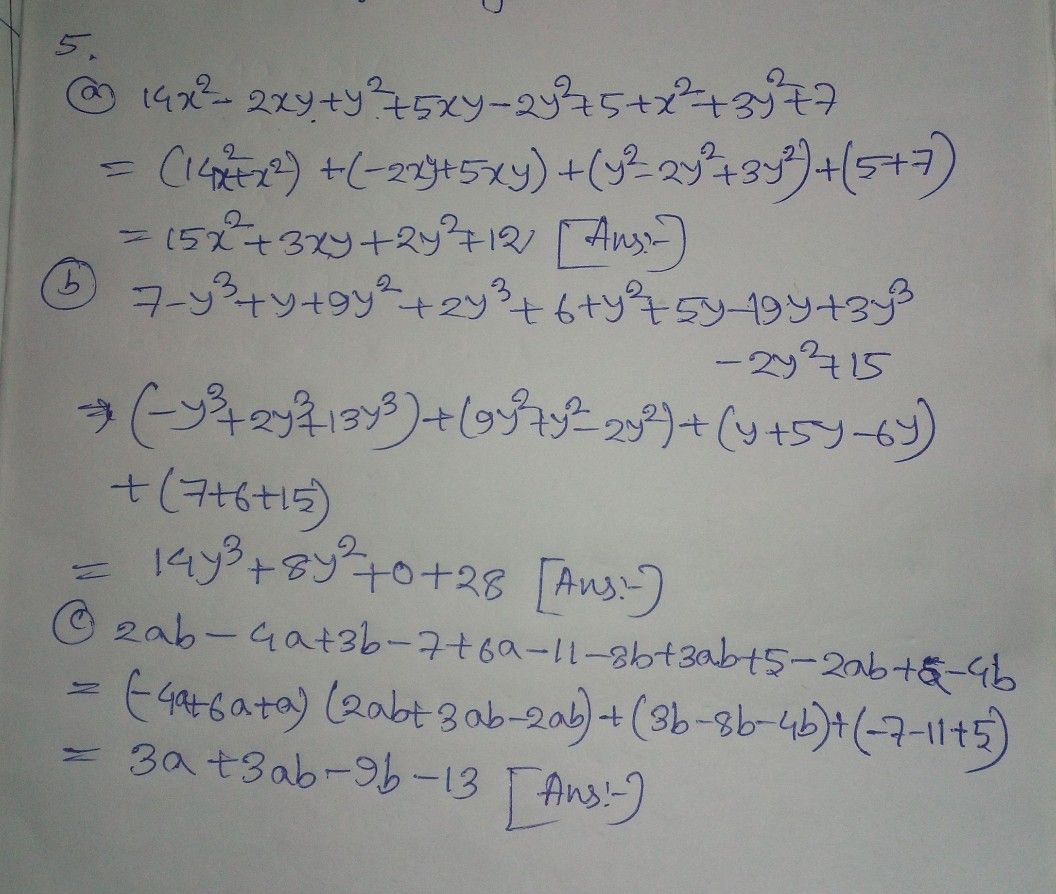Symbol
Problem$5$ Simplify: (a) $1\right)$ $14x^{2}-2xy+y^{2}+5xy-2y^{2}+5+x^{2}+3y^{2}+7$ $,$ (b) $\right)$ $7-y^{3}+y+9y^{2}+2y^{3}+6+y^{2}+5y-19y+3y^{3}-2y^{2}+15$ $\left($ (c) $2ab-4a+3b-7+6a-11$ $1-8b+3ab+5-2ab+a-4b$
7th-9th grade
Algebra
SolutionQanda teacher - sarminStudent
Excuse me
U r answer no B is wrong